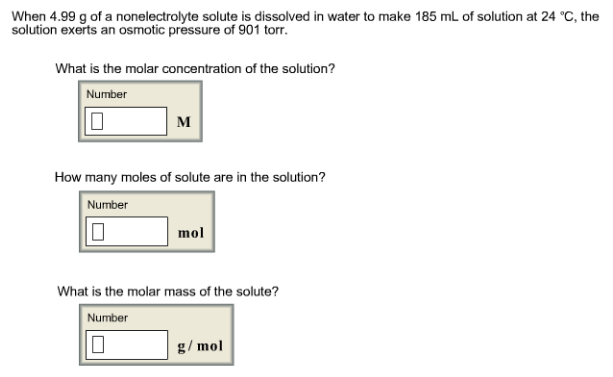# Problem: When 4.99 g of a nonelectrolyte solute is dissolved in water to make 185 mL of solution at 24°C, the solution exerts an osmotic pressure of 901 torr. What is the molar concentration of the solution? How many moles of solute are in the solution? What is the molar mass of the solute?

###### FREE Expert Solution###### Problem Details

When 4.99 g of a nonelectrolyte solute is dissolved in water to make 185 mL of solution at 24°C, the solution exerts an osmotic pressure of 901 torr.

What is the molar concentration of the solution?

How many moles of solute are in the solution?

What is the molar mass of the solute?